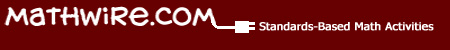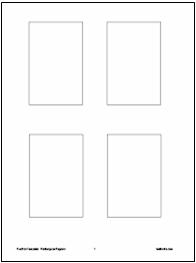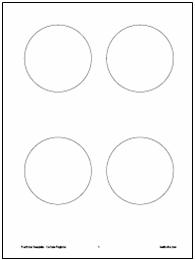# Fractions

## Fraction Templates

These templates should be inserted into sheet protectors. Students may use manipulatives or a dry-erase marker to picture fractions and solve problems.•Fraction Mats for halves, fourths, and eighths allow students to use the set model of fractions to identify how many objects are in 1/4 or 3/8 of a given number. Or, if students know how many they have altogether in the set, they can use the mats with manipulatives to figure out how much 1/4 or 5/8 of that number is.
•Fraction Circles template is useful as a pizza model.
•Fraction Rectangles template is a good, all-around fraction model that students can easily split into any number of sections.
•Fraction Squares template is also a good multi-purpose fraction model that some call the brownie model.